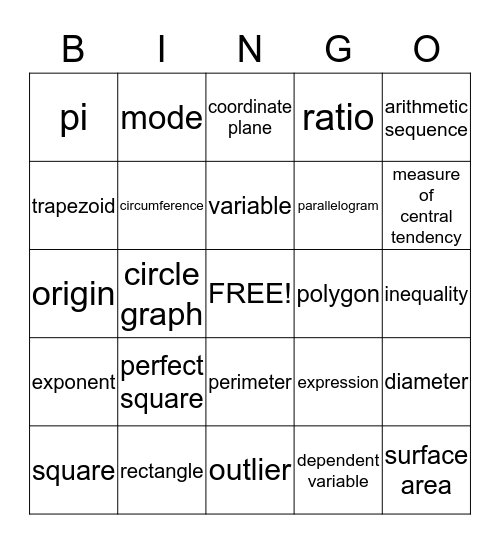# MATH REVIEW BINGOThis bingo card has a free space and 43 words: term, surface area, square root, square, rhombus, dependent variable, coordinate plane, congruent figures, arithmetic sequence, rectangle, ratio, polygon, perimeter, perfect square, percent, outlier, outcome, origin, ordered pair, measure of central tendency, mode, median, mean, pi, integer, inequality, independent variable, geometric sequence, expression, exponent, equation, diameter, coefficient, circle graph, area, whole numbers, volume, variable, trapezoid, parallelogram, quadrilateral, circumference and theoretical probability.

## Play Online